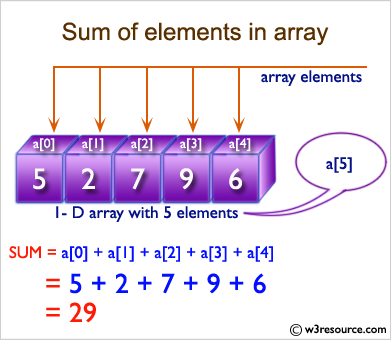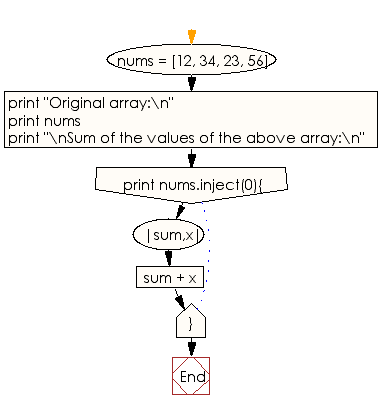﻿ Ruby Array exercises: Compute the sum of elements in a given array - w3resource# Ruby Array Exercises: Compute the sum of elements in a given array

## Ruby Array: Exercise-5 with Solution

Write a Ruby program to compute the sum of elements in a given array.Ruby Code:

``````nums = [12, 34, 23, 56]
print "Original array:\n"
print nums
print "\nSum of the values of the above array:\n"
print nums.inject(0){|sum,x| sum + x }
``````

Output:

```Original array:
[12, 34, 23, 56]
Sum of the values of the above array:
125
```

Flowchart:Ruby Code Editor: